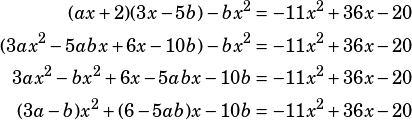If (ax+2)(3x-5b) -bx^2 = -11x^2+36x-20, what is the value of a+b? Correct ans is -1. Is it possible to solve for expression?

You’re going to want to use the fact that corresponding coefficients in equivalent polynomials must be equal. An official question would say that the equation is true for all values of x, though, which you should always see as a clue that corresponding coefficients are a way to go. FOIL out the left side.From that, you can set the following equations:

3a – b = –11
6 – 5ab = 36
–10b = –20

Start with the first one, which clearly shows you that b = 2. From there, substitute into either of the other equations to solve for a.

3a – 2 = –11
3a = –9
a = –3

If b = 2 and a = –3, then ab = –1.